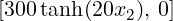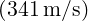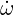### 6.4 Examples

#### 6.4.1 Wave Propagation in a Sheared Base Flow

In this section we explain how to set up a simple, 2D simulation of aeroacoustics in Nektar++. We will study the propagation of an acoustic wave in the simple case of a sheared base flow, i.e. u =T, c2 =2, ρ = 1.204kg∕m3. The geometry consists of 64 quadrilateral elements.

##### 6.4.1.1 Input file

We require a discontinuous Galerkin projection and use an explicit fourth-order Runge-Kutta time integration scheme. We therefore set the following solver information:

1<SOLVERINFO>
2    <I PROPERTY="EQType"                VALUE="APE"/>
3    <I PROPERTY="Projection"            VALUE="DisContinuous"/>
4    <I PROPERTY="TimeIntegrationMethod" VALUE="ClassicalRungeKutta4"/>
5    <I PROPERTY="UpwindType"            VALUE="LaxFriedrichs"/>
6</SOLVERINFO>

To maintain numerical stability we must use a small time-step. Finally, we set the density, heat ratio and ambient pressure.

1<PARAMETERS>
2    <P> TimeStep       = 1e-05              </P>
3    <P> NumSteps       = 1000               </P>
4    <P> FinTime        = TimeStep*NumSteps  </P>
5    <P> IO_CheckSteps  = 10                 </P>
6    <P> IO_InfoSteps   = 10                 </P>
7</PARAMETERS>

The initial condition and the base flow field are specified by the `Baseflow` and `InitialConditions` functions, respectively:

1<FUNCTION NAME="Baseflow">
2  <E VAR="u0" VALUE="300 * tanh(2*y/0.1)"/>
3  <E VAR="v0" VALUE="0"/>
4  <E VAR="c0sq" VALUE="1.4 * Pinfinity / Rho0"/>
5  <E VAR="rho0" VALUE="Rho0"/>
6</FUNCTION>
7<FUNCTION NAME="InitialConditions">
8  <E VAR="p" VALUE="0"/>
9  <E VAR="u" VALUE="0"/>
10  <E VAR="v" VALUE="0"/>
11</FUNCTION>

At all four boundaries the `RiemannInvariantBC` condition is used:

1<BOUNDARYCONDITIONS>
2  <REGION REF="0">
3    <D VAR="p" USERDEFINEDTYPE="RiemannInvariantBC"/>
4    <D VAR="u" USERDEFINEDTYPE="RiemannInvariantBC"/>
5    <D VAR="v" USERDEFINEDTYPE="RiemannInvariantBC"/>
6  </REGION>
7</BOUNDARYCONDITIONS>

The system is excited via an acoustic source termc, which is modeled by a field forcing as:

1<FORCING>
2  <FORCE TYPE="Field">
3    <FIELDFORCE> Source <FIELDFORCE/>
4  </FORCE>
5</FORCING>

and the corresponding function

1<FUNCTION NAME="Source">
2  <E VAR="p" VALUE="100 * 2*PI*5E2 * cos(2*PI*5E2 * t) * exp(-32*(x^2+y^2))"/>
3  <E VAR="u" VALUE="0"/>
4  <E VAR="v" VALUE="0"/>
5</FUNCTION>

##### 6.4.1.2 Running the code
AcousticSolver Test_pulse.xml

##### 6.4.1.3 Results

Fig. 6.1 shows the acoustic source term, the velocity and the acoustic pressure and velocity perturbations at a single time step.Figure 6.1: Acoustic source term, base flow velocity, acoustic pressure and acoustic velocity perturbations.